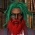Display replies flat, with oldest first Display replies flat, with newest first Display replies in threaded form Display replies in nested formRichard Schoen (University of California, Irvine), “The geometry of eigenvalue extremal problems”by Egor Pifagorov - Tuesday, 28 November 2017, 01:39 AM КОЛЛОКВИУМ ЛАБОРАТОРИИ ИМ. ЧЕБЫШЕВАЧетверг 7 декабря 17:15 ауд. 14 (14-я линия В. О., 29)Richard Schoen (University of California, Irvine) “The geometry of eigenvalue extremal problems” When we choose a metric on a manifold we determine the spectrum of the Laplace operator. Thus an eigenvalue may be considered as a functional on the space of metrics. For example the first eigenvalue would be the fundamental vibrational frequency. In some cases the normalized eigenvalues are bounded independent of the metric. In such cases it makes sense to attempt to find critical points in the space of metrics. In this talk we will survey two cases in which progress has been made focusing primarily on the case of surfaces with boundary. We will describe the geometric structure of the critical metrics which turn out to be the induced metrics on certain special classes of minimal (mean curvature zero) surfaces in spheres and euclidean balls. The eigenvalue extremal problem is thus related to other questions arising in the theory of minimal surfaces. We also discuss the question in higher dimensions. Приглашаются все желающие!